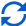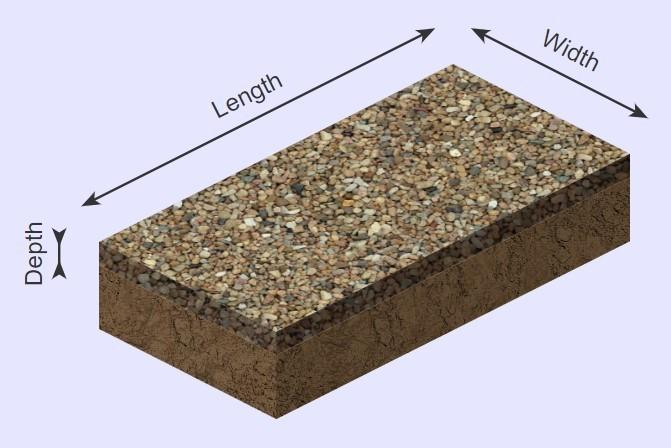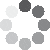Enter parameters LENGTH, WIDTH & DEPTH
Enter parameters
LENGTH, WIDTH & DEPTH
Length:
ft
• inches (in)
• feet (ft)
• yards (yd)
• centimeters (cm)
• meters (m)
Width:
ft
• inches (in)
• feet (ft)
• yards (yd)
• centimeters (cm)
• meters (m)
Depth:
in
• inches (in)
• feet (ft)
• yards (yd)
• centimeters (cm)
• meters (m)
Bag size:
ft³
• cubic inches (in³)
• cubic feet (ft³)
• cubic yards (yd³)
• cubic centimeters (cm³)
• cubic meters ()
Density:
96 - Pea Gravel lb/ft³
• Pea Gravel - 96 lb/ft³
• Custom
Price per unit of mass:
t
• pounds (lb)
• US short tons (t)
• imperial-long tons (long t)
• kilograms (kg)
Price per unit of volume:
yd³
• cubic yards (yd³)
• cubic feet (ft³)
• cubic inches (in³)
• cubic centimeters (cm³)
• cubic meters ()Result
Area:
0
ft²
• square feet (ft²)
• square inches (in²)
• square yards (yd²)
• square miles (mi²)
• acres (ac)
• square centimeters (cm²)
• square meters ()
Volume:
0
yd³
• cubic yards (yd³)
• cubic feet (ft³)
• cubic inches (in³)
• cubic centimeters (cm³)
• cubic meters ()
Weight:
0
t
• pounds (lb)
• US short tons(t)
• imperial-long tons (long t)
• kilograms (kg)
Cost:
$Bags: 0 Enter parameters AREA & DEPTH Area: ft² • square feet (ft²) • square inches (in²) • square yards (yd²) • square miles (mi²) • acres (ac) • square centimeters (cm²) • square meters () Depth: in • inches (in) • feet (ft) • yards (yd) • centimeters (cm) • meters (m) Bag size: ft³ • cubic inches (in³) • cubic feet (ft³) • cubic yards (yd³) • cubic centimeters (cm³) • cubic meters () Density: 96 - Pea Gravel lb/ft³ • Pea Gravel - 96 lb/ft³ • Custom Price per unit of mass: t • pounds (lb) • US short tons (t) • imperial-long tons (long t) • kilograms (kg) Price per unit of volume: yd³ • cubic yards (yd³) • cubic feet (ft³) • cubic inches (in³) • cubic centimeters (cm³) • cubic meters ()Result Volume: 0 yd³ • cubic yards (yd³) • cubic feet (ft³) • cubic inches (in³) • cubic centimeters (cm³) • cubic meters () Weight: 0 t • pounds (lb) • US short tons (t) • imperial-long tons (long t) • kilograms (kg) Cost:$
Bags:
0
Enter parameters
VOLUME
Volume:
yd³
• cubic yards (yd³)
• cubic feet (ft³)
• cubic inches (in³)
• cubic centimeters (cm³)
• cubic meters ()
Density:
96 - Pea Gravel lb/ft³
• Pea Gravel - 96 lb/ft³
• Custom
Bag size:
ft³
• cubic inches (in³)
• cubic feet (ft³)
• cubic yards (yd³)
• cubic centimeters (cm³)
• cubic meters ()
Price per unit of mass:
t
• pounds (lb)
• US short tons (t)
• imperial-long tons (long t)
• kilograms (kg)
Price per unit of volume:
yd³
• cubic yards (yd³)
• cubic feet (ft³)
• cubic inches (in³)
• cubic centimeters (cm³)
• cubic meters ()Result
Weight:
0
t
• pounds (lb)
• US short tons (t)
• imperial-long tons (long t)
• kilograms (kg)
Cost:
$Bags: 0(1 votes, average: 5.00 out of 5)Loading... Similar Calculators: Real-time graphics. Make the calculations and see the changes. Real-time graphics. Make the calculations and see the changes. Real-time graphics. Make the calculations and see the changes. Embed Similar Calculators:You’ll agree with us when we say that estimating how much pea gravel your next landscaping project will require is not easy! Being accurate and not wasting stones is important, as is saving money. That’s why we built our online pea gravel calculator. In this article you will see just how easy it is to use, what it calculates and which formulae it uses to do so. Using our calculator will make you far more efficient than estimating how much pea gravel you need – not to mention save you money! 💰 But first… Contents: ## Pea gravel calculator formulaBased on the measurements you provide, you can work out the area and volume of the required pea gravel using the formulae detailed below: $$Area = Length \times Width$$ $$Volume = Area \times Depth$$ $$Weight = Density \times Volume$$ Best of all… Our calculator can work out the total cost if you know the price per unit mass/volume: $$Cost = Price\,per\,unit\,mass \times Weight$$ $$Cost = Price\,per\,unit\,volume \times Volume$$ What if I don’t know the price per unit mass/volume? You can work out these values from a bag of your purchased pea gravel, using the formulae: $$Price\,per\,unit\,mass = {Total\,cost \over Total\,weight}$$ $$Price\,per\,unit\,volume = {Total\,cost \over Total\,volume}$$ Confused? 🤔 Below we show you three examples, detailing how to work out the volume, weight and cost. Otherwise, simply enter your measurements for your landscaping project! ## Example calculations • Pea Gravel Path My path measures 20 feet by 30 feet wide. I want coverage to a depth of 3 inches with normal pea gravel (96 lb/ft³) costing$45 per US short ton.

I simply enter these measurements to do the following operations:

$$Area = Length \times Width = 200\,ft \times 300\,ft = 600\,ft^2$$

$$Volume = Area \times Depth = 600\,ft^2 \times 3\,in = 5.556\,yd^3$$

$$Weight = Density \times Volume = 96\,lb/ft^3 \times 5.556\,yd^3 = 14,400\,lb$$

$$Cost = Price\,per\,unit\,mass \times Weight = 45\,/t \times 14,400\,lb = 324$$

• Cover 0.1 Cubic Yards of Driveway Calculating Price Per Unit Volume

I can purchase 10 cubic feet for a cost of $300 and I want 0.1 cubic yards for my path. $$Price\,per\,unit\,volume = {Total\,cost \over Total\,volume} = {300 \over 10\,ft^3} = 30\,/ft^3$$ I then calculate: $$Weight = Density \times Volume = 96\,lb/ft^3 \times 0,1\,yd^3 = 0,31\,t$$ $$Cost = Price\,per\,unit\,volume \times Volume = 30\,/ft^3 \times 0,31\,t = 81$$ At this point, you may be asking: What if I don’t know the density of the material? You can calculate density using the formula below and enter your value in the custom field: $$Density = {Mass \over Volume}$$ • Custom Aggregate Example I want to cover 10 ft² of path to a depth of 5 in with gravel weighing 200 lb per 4 cubic feet and costing$45 for each US short ton.

$$Density = {Mass \over Volume} = {200\,lb \over 4\,ft^3} = 50\,lb/ft^3$$

$$Volume = Area \times Depth = 10\,ft^2 \times 5\,in = 0.154\,yd^3$$

$$Weight = Density \times Volume = 50\,lb/ft^3 \times 0.154\,yd^3 = 0.104\,t$$

$$Cost = Price\,per\,unit\,mass \times Weight = 45\,/t \times 0.104\,t = 4.69$$

At this point, it’s likely that you are asking the following question:

## What if my measurements are in different units?

You might notice that we changed between units (e.g. Density is in pounds per cubic feet and volume is given in cubic yards).

The calculator does the following conversions for you:

$$1\,ft = 12\,in = 0.33\,yd = 30.48\,cm = 0.3048\,m$$

$$1\,t = 2000\,lb = 0.893\,long\,t = 907\,kg$$

We think that our pea gravel calculator is a powerful online tool that can help you find out how many bags of gravel you need, eliminating wasted stones, and of course save you money!

Embed this calculator on your site!Add live graphics
Copied to clipboard! Preview